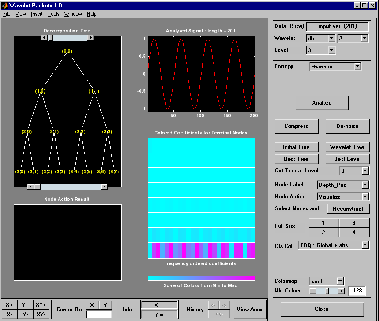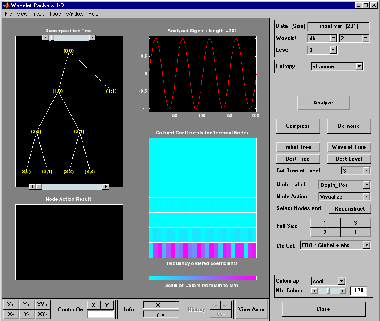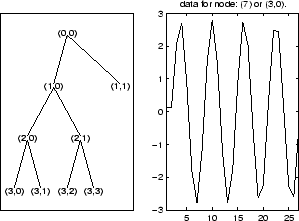Read wavelet packet decomposition tree from figure

## Syntax

```T = readtree(F) ```

## Description

`T = readtree(F)` reads the wavelet packet decomposition tree from the figure whose handle is `F`.

## Examples

```% Create a wavelet packet tree. x = sin(8*pi*[0:0.005:1]); t = wpdec(x,3,'db2'); % Display the generated tree in a Wavelet Packet 1-D GUI window. fig = drawtree(t); ``````%------------------------------------- % Use the GUI to split or merge Nodes. %------------------------------------- ``````t = readtree(fig); plot(t) % Click the node (3,0), (see the `plot` function). ```## Version History

Introduced before R2006a